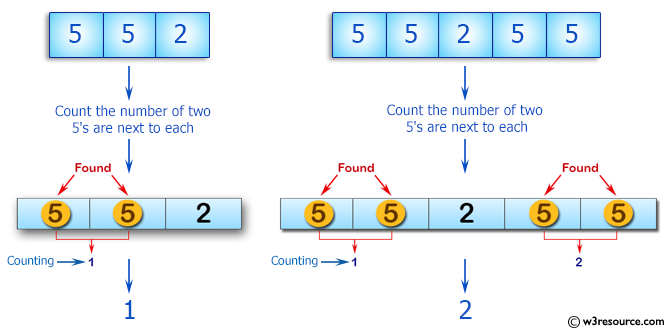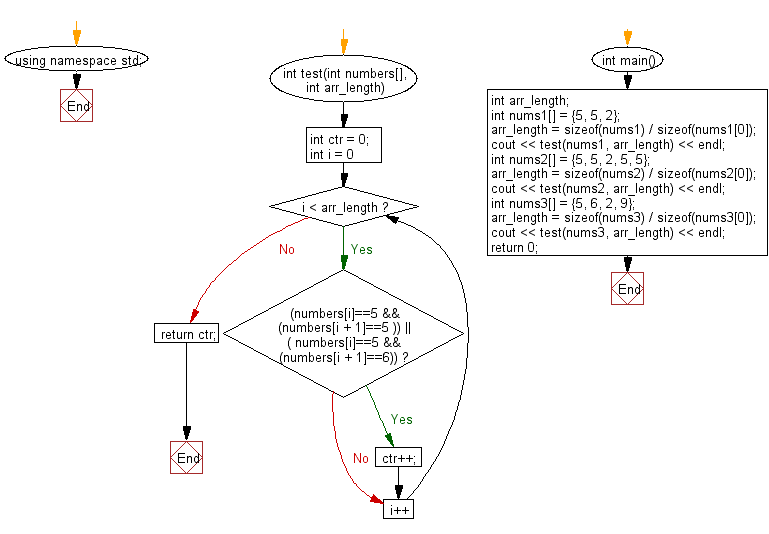﻿ C++ : Number of two 5s are next to each other in an array# C++ Exercises: Count the number of two 5's are next to each other in an array of integers

## C++ Basic Algorithm: Exercise-35 with Solution

Write a C++ program to count the number of two 5's are next to each other in an array of integers. Also count the situation where the second 5 is actually a 6.

Sample Solution:

C++ Code :

``````#include <iostream>

using namespace std;

int test(int numbers[], int arr_length)
{
int ctr = 0;
for (int i = 0; i < arr_length; i++)
{
if ((numbers[i]==5 && (numbers[i + 1]==5 )) || ( numbers[i]==5 && (numbers[i + 1]==6))) ctr++;
}
return ctr;
}

int main()
{
int arr_length;
int nums1[] = {5, 5, 2};
arr_length = sizeof(nums1) / sizeof(nums1);
cout << test(nums1, arr_length) << endl;
int nums2[] = {5, 5, 2, 5, 5};
arr_length = sizeof(nums2) / sizeof(nums2);
cout << test(nums2, arr_length) << endl;
int nums3[] = {5, 6, 2, 9};
arr_length = sizeof(nums3) / sizeof(nums3);
cout << test(nums3, arr_length) << endl;
return 0;
}
``````

Sample Output:

```1
2
1
```

Pictorial Presentation:Flowchart:C++ Code Editor:

Contribute your code and comments through Disqus.

What is the difficulty level of this exercise?

﻿